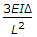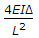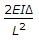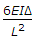# Civil Engineering - UPSC Civil Service Exam Questions

26.

Which one of the following statements is correct ? Compared to IFR conditions, the runaway capacity of a runway operating under VFR consitions

 A. is higher B. is lower C. is the same D. cannot be generalised

Explanation:

No answer description available for this question. Let us discuss.

27.

In an otherwise symmetrical portal frame with one end fixed and the other end hinged, the large support sinks by an amount Δ. The fixed and bending moment induced at each end of the horizontal member of the frame due to the sinking of the support will be (given that 'L' is the length of the member and 'E' is the flexural stiffness)

 A.B.C.D.Explanation:

No answer description available for this question. Let us discuss.

28.

The total observed runoff volume during a 4 hour storm with a uniform intensity of 2.8 cm/hr is 25.2 x 106 m3 from a basin of 280 km2 area. What is the average infiltration rate for the basin ?

 A. 3.6 mm/hr B. 4.8 mm/hr C. 5.2 mm/hr D. 5.5 mm/hr

Explanation:

No answer description available for this question. Let us discuss.

29.

A car accelerates from rest to a speed of 10 m/s and the energy spent in doing this is E. If the car is further accelerated from 10 m/s to 20m/s, what is the amount of energy to be spent further ?

 A. E B. 2E C. 3E D. 4E

Explanation:

No answer description available for this question. Let us discuss.

30.

The losses in prestress in pre-tensioning system are due to :
1. elastic deformation of concrete when wires are tensioned successively
2. friction
3. shrinkage and creep of concrete
Select the correct answer using the codes given below:

 A. 1, 2 and 3 B. 2 and 3 C. 1 alone D. 3 alone